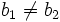# Snevily's conjecture for subsets of size two

## Statement

Suppose$G$ is an odd-order abelian group and$A = \{ a_1, a_2 \}$ and$B = \{ b_1, b_2 \}$ are (not necessarily disjoint) subsets of$G$ of size two. Then, one of these is true:$a_1 + b_1 \ne a_2 + b_2, \qquad a_1 + b_2 \ne a_2 + b_1$.

## Facts used

1. kth power map is bijective iff k is relatively prime to the order

## Proof

Given: A finite Abelian group$G$ of odd order, subsets$A = \{ a_1, a_2 \}$ and$B = \{ b_1, b_2 \}$ of$G$.

To prove: Either$a_1 + b_1 \ne a_2 + b_2$ or$a_1 + b_2 \ne a_2 + b_1$.

Proof: Suppose equality holds in both cases. Then, subtracting the two equations, we get:$(a_1 + b_1) - (a_1 + b_2) = (a_2 + b_2) - (a_2 + b_1)$.

Rearranging this, we get:$2(b_1 - b_2) = 0$.

Since$b_1 \ne b_2$,$b_1 - b_2 \ne 0$, and since the group is Abelian of odd order, its double is also therefore nonzero (using fact (1)).